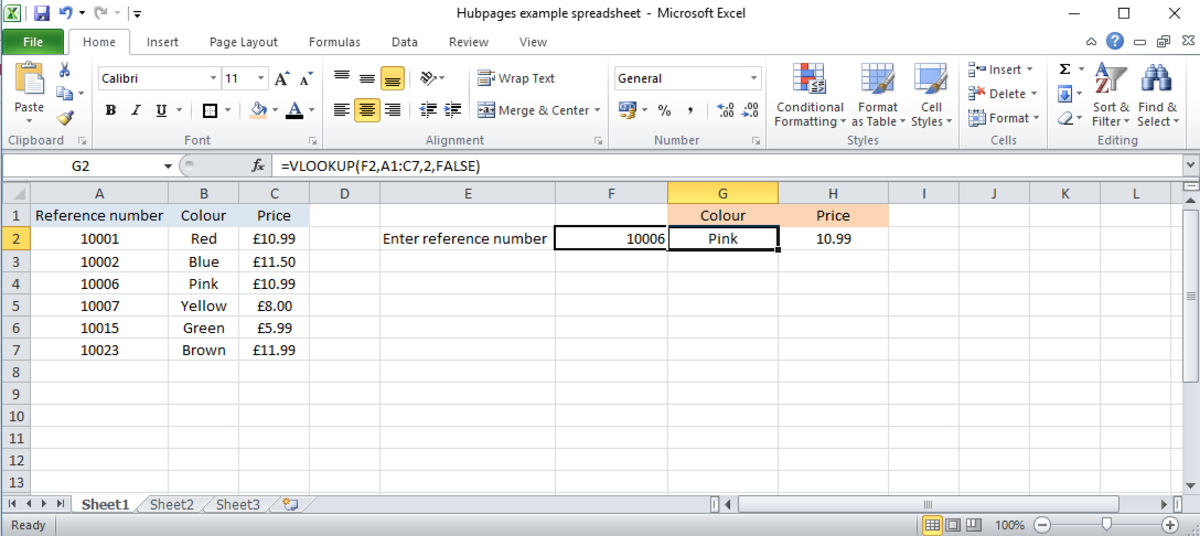# How to Use the VLookup Function in Microsoft Excel

• Author:
• Updated date:How to Use the VLOOKUP function in Microsoft ExcelMy own screen print

## Using the VLOOKUP function

The VLOOKUP function is an extremely helpful tool in Microsoft Excel. It searches for a value in a table and then returns a corresponding value from the same row.

In the example in this article, I have set up a basic database for a hardware store that sells 6 different colours of paint. By using the VLOOKUP Function, we can type the paint's reference number into Excel and the colour and price of the paint will be returned to us automatically.

## Syntax

The syntax for the VLOOKUP formula is as follows:

=VLOOKUP(lookup_value, table_array, col_index_num, [range_lookup])

This looks confusing to start with, but once you get used to it, it is actually very easy to use.

Let's look at each part as it is used in the hardware store example.

## Lookup value

The 'Lookup value' denotes the box containing the data that you want the VLOOKUP function to search for. In my example, I want to enter a reference number into box F2 and have the colour of the paint returned in box G2.

I enter "=VLOOKUP" into box G2 and for my Lookup value I enter "F2". This means that Excel knows to look at the value in F2.

We now need to tell Excel what to compare the F2 value to. This is where the next part comes in.

## Table

I want Excel to take the reference number I have typed into F2 and find it in the table circled in the picture. To tell Excel to do this I need to type the reference for the table into the second part of the VLOOKUP formula.

If you have given your table a name, you can type this into the formula, but in my example I have just given the range of the table A1:C7.

So far our formula looks like this - =VLOOKUP(F2,A1:C7.

We now need to tell Excel where to find the value that corresponds to our reference number in F2. This is what the index number is for.

## Index Number

The third part of the formula is the index number. This tells Excel which column of the table to take the corresponding value from.

I have entered =VLOOKUP(F2,A1:C7,2,... This tells Excel to look for my reference number in the table A1:C7 and then return the value from the same row as my reference number, but in the second column. In this example, Excel has found '10006' in the table and returned the value from the second column, which is 'Pink'.

In box H2, I have entered =VLOOKUP(F2,A1:C7,3, hence Excel has returned the value from the third column, which is the price '10.99'.

## Range Lookup

The Range Lookup part of the formula is where you tell Excel how accurate you want the returned data to be. You have two options to enter:

• False: Excel will search for exactly what you have typed. If it cannot find an exact match, then it will return 'N/A'.
• True: Excel will search for an approximation to what you have typed.

In this example I have put 'false' so if I type 10004, Excel returns 'N/A' as there is no exact match in the table.

If I had used 'true' instead, Excel would pick the next number available below 10004, which in this example is 10002. It would then return the corresponding value for 10002, which is 'blue'.

## Final Formula in Full

My full formula in box G2 is =VLOOKUP(F2,A1:C7,2,FALSE).

This takes the input from F2, looks for it in the table A1:C7 and returns the value from the same row as my input, but in the second column (the paint colour column).

In box H2 I have the almost identical formula =VLOOKUP(F2,A1:C7,3,FALSE) so Excel now returns the value from the third column of the table (the price column).

As I have used 'false' in my formula, Excel will return 'N/A' if the exact number I type in cannot be found.

## Extra information

The VLOOKUP function is not just confined to within one sheet in your Excel document. You can direct it to look for information within other sheets or even in another Excel document. As long as you enter the reference for the table into the second part of the formula, Excel will find it.